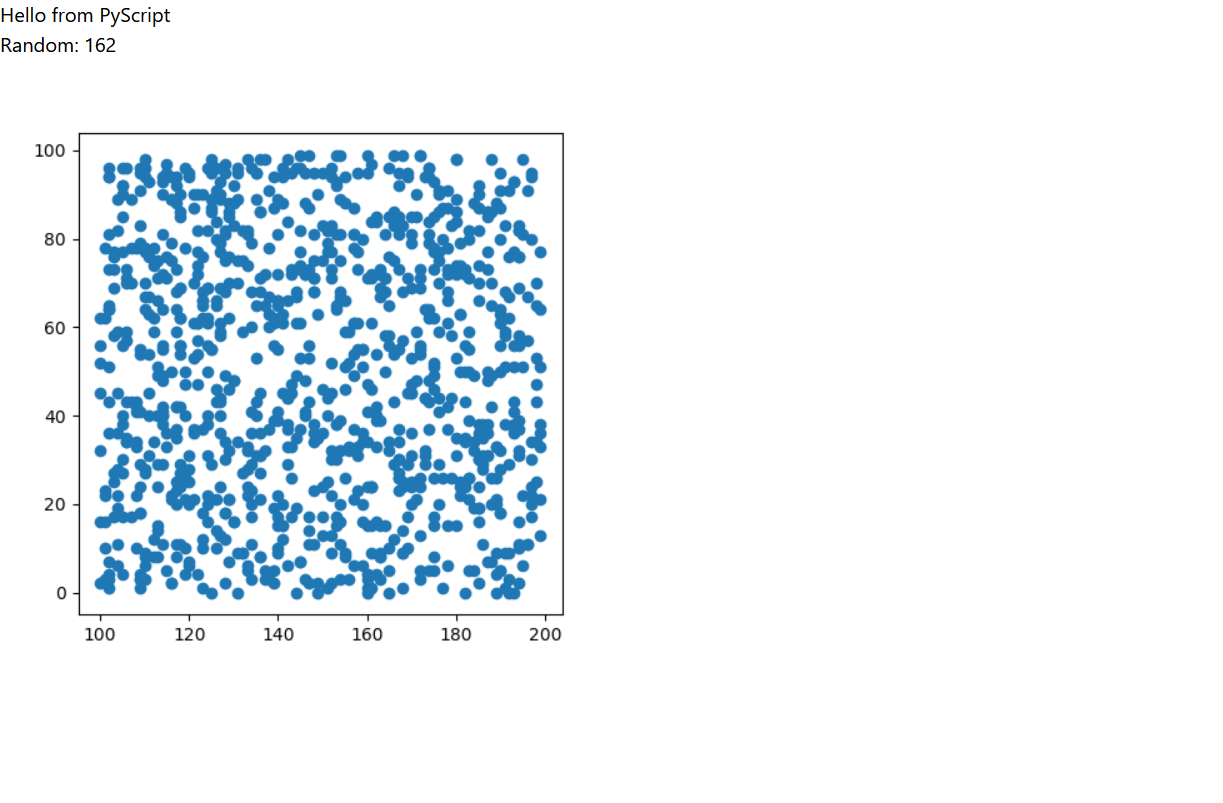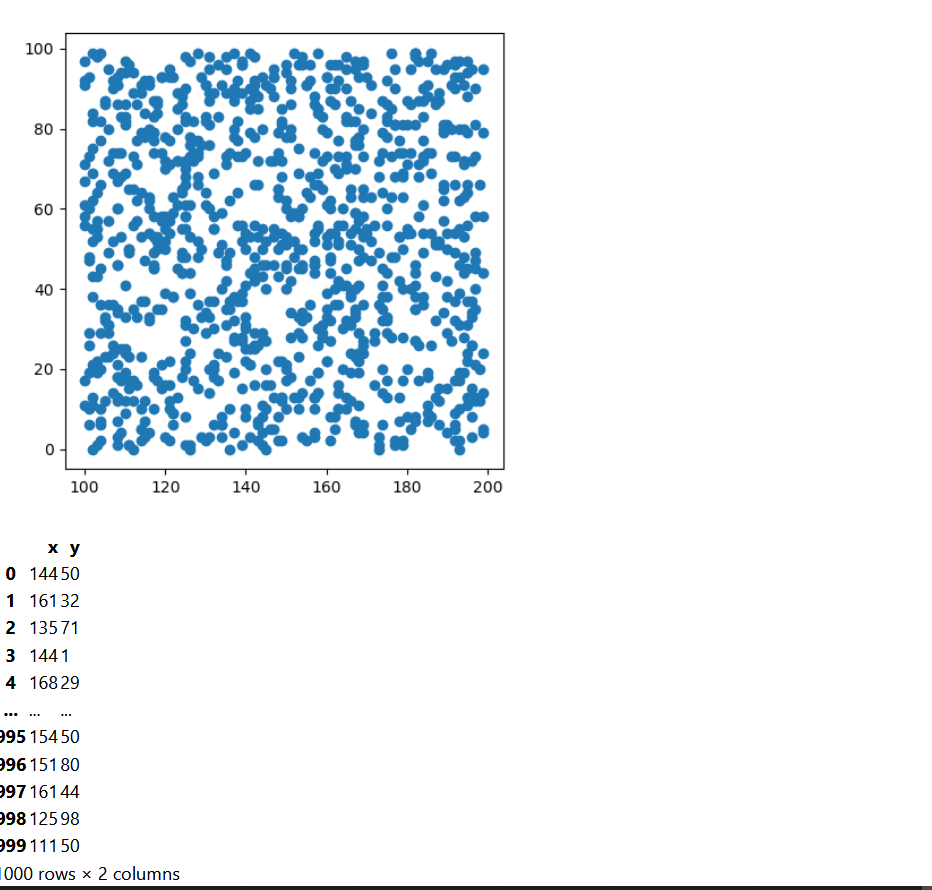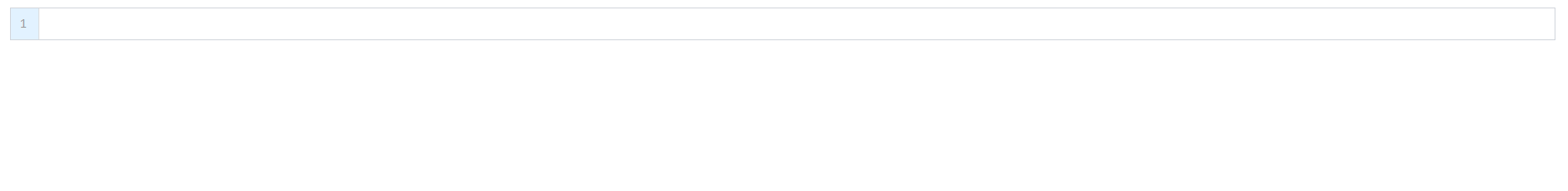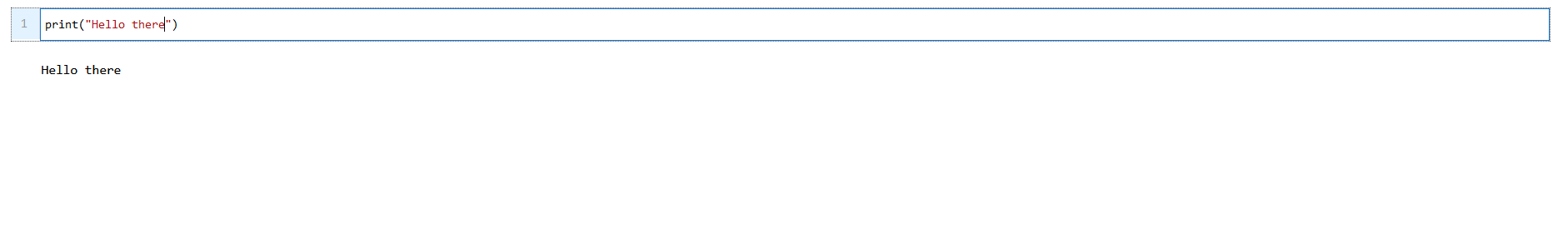# Pyscript: Running Python in Webpages

## Introduction

Hello and whats up everyone, in this blog we will explore PyScript for running Python codes inside our HTML files. It is quite easy to do so. How does it works under the hood is not what is being focused here but what can we do will be. For docs, please visit here.

## First Program

• Create a HTML file and on the top, import packages inside the head section. html
PyScript Test

# print("Hello from PyScript")


Opening above HTML in browser will show like below:

![](https://q-viper.github.io/assets/pyscript/hello.png)

## Importing Default Package

Lets add below code just below above's to print random number generated between 100 and 200.

html
<py-script>
import random
print(f"Random: {random.randint(100,200)}")
</py-script>


Output will be something like below:## Importing External Libraries

Lets import NumPy. It is not possible to import them by default as they are not installed. Hence we need to include them in environment section like below.

<html>
<title>PyScript Test</title>
<script defer src="https://pyscript.net/alpha/pyscript.js"></script>

<body>
<py-env>
- numpy
- sympy
- matplotlib
</py-env>

<py-script>print("Hello from PyScript")</py-script>
<py-script>
import random
print(f"Random: {random.randint(100,200)}")
</py-script>
<py-script>
import numpy as np
import matplotlib.pyplot as plt

x = np.random.randint(100,200,1000)
y = np.random.randint(000,100,1000)
fig = plt.figure(figsize=(5,5))
plt.scatter(x,y)
fig
</body>
</html>


It will take little bit to see the result like below:### Pandas Dataframe

Just like we used NumPy above, we can include pandas too and lets see it in action.

<html>
<title>PyScript Test</title>
<script defer src="https://pyscript.net/alpha/pyscript.js"></script>

<body>
<py-env>
- numpy
- sympy
- matplotlib
- pandas
</py-env>

<py-script>print("Hello from PyScript")</py-script>
<py-script>
import random
print(f"Random: {random.randint(100,200)}")
</py-script>
<py-script>
import numpy as np
import matplotlib.pyplot as plt

x = np.random.randint(100,200,1000)
y = np.random.randint(000,100,1000)
fig = plt.figure(figsize=(5,5))
plt.scatter(x,y)
fig
</py-script>
<py-script>
import pandas as pd
df = pd.DataFrame({"x":x,"y":y})
df
</py-script>
</body>
</html>## Live Code Editor

We can even have our own code editor in browser using PyScript. It can be done by using tag <py-repl>. Lets see it in action.

<html>
<title>PyScript Test</title>
<script defer src="https://pyscript.net/alpha/pyscript.js"></script>

<body>
<py-env>
- numpy
- sympy
- matplotlib
- pandas
</py-env>
<py-repl>
</body>
</html>


We can see something like below in a browser:What is interesting is that we can run code by shift+enter just like in Jupyter Notebook.Tags:

Categories:

Updated: